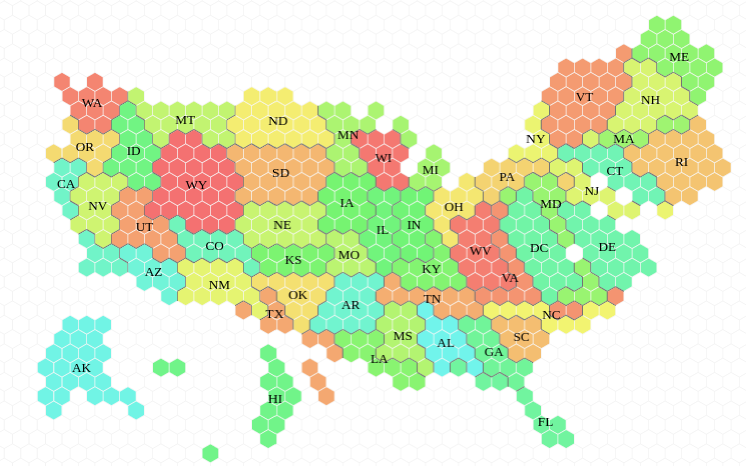Riddler and Voter Power Index

Oliver Roeder has a nice puzzle: the riddler. Just like last week, this week’s puzzle has an interesting application to the US Election and I enjoyed it really much, so I figured I might just write a blog post 🙂 In this article, we’ll solve this week’s riddler two different ways (just because :p) and discuss an indicator used on FiveThirtyEight’s prediction model for the election: the Voter Power Index.

Exact solution and Stirling approximation

I won’t write again the problem and notations, but you can find them here. We’ll also assume N is odd (as precised later by Ollie on Twitter). This assumption won’t matter much because we’ll only look at applications for large values of N. Let’s write:

$$\mathbb{P} = \Pr(you~decide~the~election)$$

Your vote is obviously going to be decisive if there is a tie between the N-1 other votes (convienently, N-1 is even). The votes are all independant with same probability p=1/2, so they are Bernoulli trials. Consequently, the probability we’re looking for is the probability that exactly half of these Bernoulli trial succeed, which is by definition the binomial distribution. Thus:

$$\mathbb{P} = {{N-1}\choose{\frac{N-1}{2}}} p^{\frac{N-1}{2}} {(1-p)}^{\frac{N-1}{2}}$$

As p=0.5, the exact value for the probability of your vote being decisive is thus:

$$\fbox{\mathbb{P} = \frac{{{N-1}\choose{\frac{N-1}{2}}}}{{2}^{N-1}}}$$

So, here is the exact solution, but it’s not super useful as is. Much more interesting is how this varies with N (with N sufficiently large). We can use Stirling’s approximation:

$$\log \mathbb{P} = \log {{N-1}\choose{\frac{N-1}{2}}} – (N-1) \log 2 \\ ~~~~\sim N \log N – \frac{N}{2} \log \frac{N}{2} – \frac{N}{2} \log \frac{N}{2} + \frac{1}{2} \left( \log N – \log \frac{N}{2} \\~~~~~~~- \log \frac{N}{2} – \log 2\pi \right) – N \log 2 \\~~~~\sim – \frac{1}{2} \log N + \log 2 – \frac{1}{2} \log 2\pi$$

Thus for sufficiently large N, the probability your vote is the decisive vote varies like the inverse of the square root of N:

$$\fbox{\mathbb{P}\sim \sqrt{\frac{2}{N\pi}} \approx \frac{0.8}{\sqrt{N}}}$$

A very simple solution for large N

Actually, we could have obtained this result for large N much more simply. We know that asymptotically the binomial distribution is gonna converge to a normal distribution. The event that your vote is the decisive one is actually the most probable event, as probabilities that the other people vote for either candidates are equal to 1/2. So the solution to the riddler can be easily computed using the density of the normal distribution:

$$\mathbb{P} = \phi(0) = \frac{1}{\sqrt{2\pi \sigma^2}}$$

with:

$$\sigma^2 = Np(1-p) = \frac{N}{4}$$

(the variance of the binomial distribution), we get the same result as in the first paragraph:

$$\fbox{\mathbb{P}\sim \sqrt{\frac{2}{N\pi}} \approx \frac{0.8}{\sqrt{N}}}$$Mode of normal distribution for various standard deviations. © W. R. Leo

Voter Power Index

$$\sqrt{\frac{510000}{150000}} \approx 1.8$$